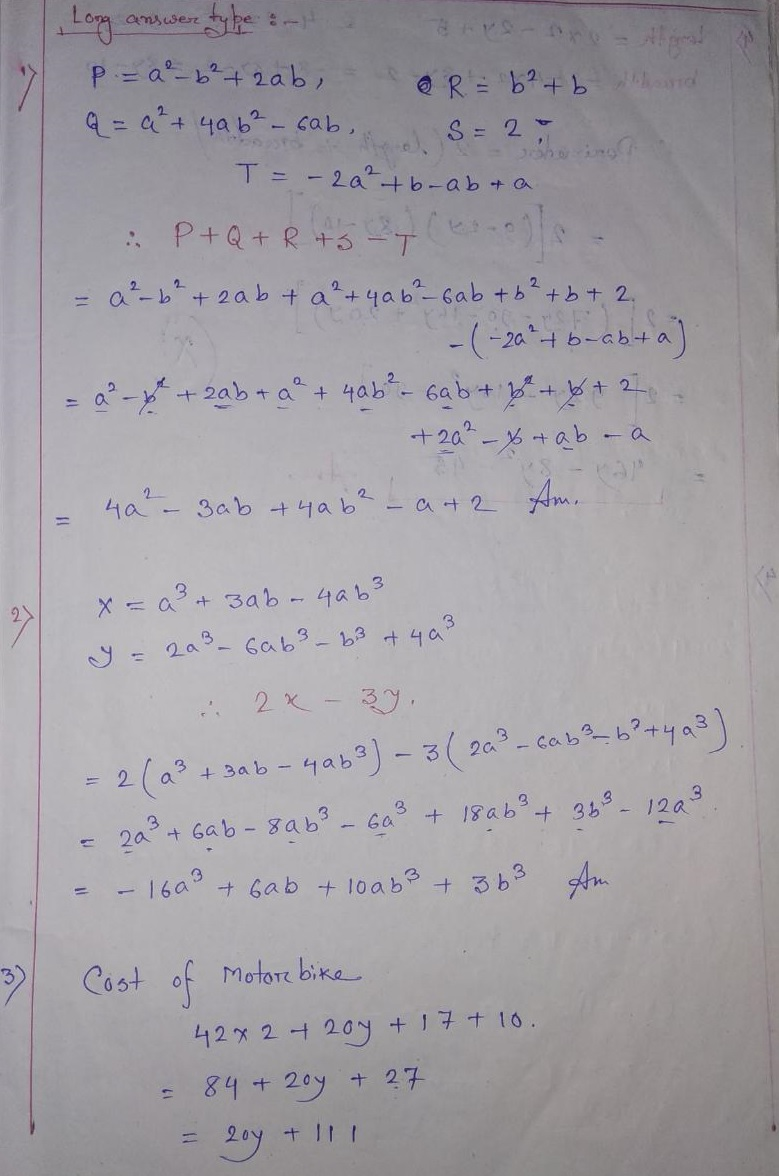# Algebraic Expressions Extra Questions Solution for Class 7

## Algebraic Expressions Class 7 Mathematics Extra Question and Answers for CBSE / NCERT Board Students

Algebraic Expressions Extra Questions and Answers for Class 7 Level Students of CBSE / NCERT Board Published in this Page. Here we provided 01 Marks that’s mean MCQ Type Questions and their Solution And Long Answer Type Questions & Solution. Class VII Students can follow this Page.

### Long Answer Type Extra Questions

(1) If P = a2 – b2 + 2ab, Q = a2 + 4ab2 – 6ab, R = b2 + b, s= 2 T = -2 a2 + b – ab + a, find P + Q + R + S – T.

(2) If x = a3 + 3ab – 4ab3 andy = 2a3 – 6ab3 – b3 + 4a3 than determine 2x – 3y

(3) Amit sold motorbike for Rs 42×2 + 20y + 17. If the loss is Rs 10, what is the cost of motorbike ?

(4) The length and breadth of a rectangular are 2×2 – 2y + 5 and -4×2 + 8y-2. Find the perimeter of the rectangle. What will be the perimeter of rectangle if x = 1  And y = 2 ?

Solution:### MCQ Type

(1) If m= -2, value of 4m3 + 2m2 – 10 is

(a) -32

(b) – 34

(c) 40

(d) 30

(2) Sum of 3m and 2n is

(a) 5mn

(b) 3m+2n

(c) 5m

(d) 5n

(3) The number of terms in 4p2q – 3qp2 + 5is

(a) 2

(b) 3

(c) 5

(d) 1

(4) The expression for sum of numbers a and b subtracted from their products is

(a) a + b-ab

(b) ab – a+b

(c) ab – (a+b)

(d) ab + a-b.

In case you are missed :- Previous Chapter Extra Questions

(5) Algebraic expression x2 + 5x2 is a

(a) Monomial

(b) binomial

(c) Trinomial

(d) None of the above

### Short Answer Type  Extra Questions

(1) Rashi bought an item for Rs xy – 2xy2, Siya bought another item and paid Rs 2xy2 + x2 more than Rashi. Find the amount paid by siya

(2) Verify : (x-y)2 = x2 – 2xy + y2 for x = 4, y = 1.

(3) Multiply these algebraic expressions :

(m – 2n ) (mn2 – 2m + n )

(4) write the terms and factors of : – 4x3 y – 4x2y2

(5) Subtract the sum of  x-4xy + z and 2xy + 2xy + 2z -4x from the sum of -6x + 4z and -18 z.

Solution:### Very Short Answer Type Extra Questions

(1) Find the value of the following expression for a = 3, b=2; 7a -4b

(2) Subtract 4a – 7ab + 3b + 12 from 12 a – 9ab + 5b – 3

(3) Add 7xy + 5yz – 3xz; 4yz + 9zx – 4y, -3xz + 5x – 2xy.

(4) When 2x-4x2y2+1 taken away from P, the resulting expression is 4x + x2y2 -3. Find P

(5) The sum of two algebraic expressions is 6x3 – 2xy + 4y2, if one of the expressions is 4xy – 2y2 then find the other expression.

Solution:Share Us to Your School Friends…

In case you are missed :- Next Chapter Extra Questions

Updated: April 5, 2023 — 4:55 pm